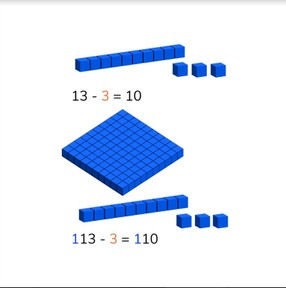Subtraction to 1,000 via simplification

# Subtraction to 1,000 via simplification

No account needed.8,000 schools use Gynzy92,000 teachers use Gynzy1,600,000 students use Gynzy

## General

Students learn that you can use a simplified subtraction problem to use as a first step to solving a more difficult subtraction problem. If you know the difference of a subtraction problem without the hundreds, you can quickly determine the answer with hundreds.

2.NBT.B.7

## Relevance

It is important to learn this to become better at quickly and easily solving more difficult subtraction problems.

## Introduction

Start the lesson by composing numbers from three given digits. You can compose 6 different numbers with the digits given. Ask students to work in groups to see if they can come up with all 6 numbers. Then ask students what the highest and lowest numbers are that you can create. Finally give students a pop quiz with subtraction problems to 100 with and without regrouping.

## Development

Show students a set of subtraction problems in which simplification is shown. Ask students if they notice anything about the set of subtraction problems. They should notice that the answers all have hundreds added to them. They can also notice that the subtraction problems are linked- that they are part of the same set. Tell the students that they can solve an subtraction problem with hundreds easier if they first solve the subtraction problem without the hundreds. You can use that as your first step. If you can solve 6 - 2 = 4, then you can also solve 106 - 2 = 104 and 206 - 2 = 204 without needing to recalculate the entire problem. Show this using the MAB-blocks on the interactive whiteboard. Check that students are able to solve a subtraction problem with visual support. First 13 - 3, then 113 - 3. Next move to a few subtraction problems in which the students don't have visual support. Remind students that they don't need to recalculate each problem because they simply add the hundreds to the first step they solved without the hundreds. Show students a set of subtraction problems and ask them to explain how to solve them.

Check that students are able to subtract numbers to 1,000 using simplification by asking the following questions:
- 7 - 2 = 5, what is 107 - 2?
- 14 - 3 = 11, 214 - 3 = ? How do you know?
- 58 - 19 = 39. Which subtraction problem can you now also easily solve?

## Guided practice

Studenst are given subtraction problems to solve using simplification. They are given subtraction problems with visual support, without visual support, with and without regrouping. They are also given sets of subtraction problems to solve.

## Closing

Remind students why it is important to be able to use simplification. By learning to simplify, they learn to make these problems easier to solve. If you can solve the subtraction problem without the hundreds, you can also solve the subtraction problem with the hundreds. Check that students understand by solving a few sets of subtraction problems individually or as a class.

## Teaching tips

Students who have difficulty can be supported by making use of MAB blocks or using money. If you give a student a 10 dollar bill and a 20 dollar bill(30 dollars in total). You know that 30 - 20 = 10. If you give them a 100 dollar bill, they now have 130 - 20 = 110. With another 100 dollar bill the problem becomes 230 - 20 = 210. Repeat with different amounts. Emphasize that they don't need to recalculate the entire problem because they already solved the first step.

## Instruction materials

Optional: MAB blocks/manipulatives or money

### The online teaching platform for interactive whiteboards and displays in schools

• Save time building lessons

• Manage the classroom more efficiently

• Increase student engagement